AP Chemistry : Acid-Base Reactions

Example Questions

← Previous 1 3 4 5 6 7 8 9 10

Example Question #1 : P H

What is the pH of a solution that has [OH-] 1 X 10–4 M?

-4

6

4

8

10

10

Explanation:

pOH would be 4 (use –log [OH–]) and pH would be 14–pOH = 14 – 4 = 10

Example Question #2 : P H

What is the pH of a solution with [OH-] = 4 X 10-6

6

4

8.6

2.2

12.1

8.6

Explanation:

[OH-] = 4 X 10-6

pOH = 5.4 — use –log [OH] to find pOH

pH = 14– pOH = 8.6

Example Question #3 : P H

What is the pH of a soution containing .0001 M HCl?

4

3

7

5

6

4

Explanation:

The pH of a solution is determined by taking the negative log of the concentration of hydrogen ions in solution.  HCl is strong acid so it completely dissociates in solution.  So adding .0001 M HCl is the same as saying that 1 *10-4 moles of H+ ions have been added to solution.  The -log[.0001] =4, so the pH of the solution =4.

Example Question #4 : P H

Which of the following solutions contains the greatest number of H+ ions?

5 mL of NaOH

0.010 mL of KOH in excess water

1.0 mL of NaCl in excess water

0.010 mL of HCl in excess water

0.010 mL of CH3OH in excess water

0.010 mL of HCl in excess water

Explanation:

This question asks for the solution with the greatest number Hions, we can also approach this problem as if we are looking for the solution with the lowest pH. HCl is strong acid, therefore it will dissociate completely in solution. So the solution containing 0.010 mL of HCL contains 0.010 mL of Hions in solution, in addition to the H+ ions that are already in solution due to the auto-ionization of water. The only other solution that could have a pH less than 7 would be the one with 0.010 mL of CH3OH in excess water, becasue CH3OH is very slightly acidic.  But since it is compared with an equal volume of HCl which is strong acid, it can be said that the most Hions will be found in the solution containing a small amount of strong acid, HCl.

Example Question #5 : P H

What is the approximate pH of a 1.0 M solution of soluble CaCO3?

2

8

4

7

8

Explanation:

Calcium carbonate is a base since it's the salt of a strong base (NaOH) and a weak acid (carbonic acid). The only basic pH on the list is 8, making it the correct answer.

Example Question #6 : P H

What is the pH of a 1 * 10–3M  solution of H2CO3 acid? (pKa is 6.4)

3.2

8.8

4.7

5.6

4.7

Explanation:

The –log of the pKa will give you the Ka, so take the –log (6.4), which gives you approximately 4 * 10–7. The Ka expression is set up with products over reactants (hydrogen carbonate ion * hydrogen ion/carbonic acid). The undissociated carbonic acid is 0.001M, and you should use the variable 'x' to account for how much it dissociates and how many of the ions are produced. Ka = 4 * 10–7 = x2/0.001 ends up being your Ka expression, if you assume x is negligible compared to the original concentration of 0.001. Solving for x, you get 2 * 10–5. This is the hydrogen ion concentration. pH = –log (hydrogen ion concentration), so pH = –long(2 * 10–5), which is approximately 4.7.

Example Question #7 : P H

What is the range of possible hydrogen ion concentrations in acidic solution?

7

1 to 7

10–13 to 10–7.001

10–1 to 10–6.999

13 to 14

10–1 to 10–6.999

Explanation:

For a solution to be acidic, it must have a pH between 1 and 6.99, since 7 is neutral; pH is –log(hydrogen ion concentration), the range of possible values is between 10–1 and 10–6.999.

Example Question #8 : P H

What is the pH of a 0.05M solution of hydroflouric acid?

3.0

7.0

5.0

4.5

3.0

Explanation:

The Ka = [H+][F–]/[HF]. The beginning concentration of HF is given as 0.05, and we can use x as the variable that accounts for how much of the HF is lost, along with how much of the H+ and F– are formed.

Thus, Ka = x2/.05 if you use the approximation that x is negligible compared to the starting concentration of HF.

Solving for x, x = 1 * 10–3. This is the H+ ion concentration.

pH = –log(H+) = –log(1.0 * 10–3)) = 3

Example Question #9 : P H

Which of the following will produce the solution with the lowest pH?

0.2M HCl

0.3M HI

0.5M HF

2.0M NaOH

0.3M HI

Explanation:

NaOH is a base, so that won't produce an acidic solution. Of the remaining acids, HCl and HI are strong acids, and HF is weak. HI is at a higher molarity, so it will produce the most acidic solution.

Example Question #10 : P H

A chemist adds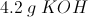to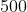mL of water. What is the pH of the solution?

0.8248

0

7.00

14.0

13.18

13.18

Explanation:

First, we will calculate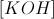as follows: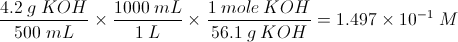Now we will calculate the pOH: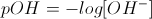In solution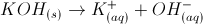, so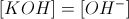.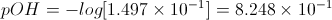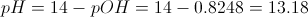← Previous 1 3 4 5 6 7 8 9 10